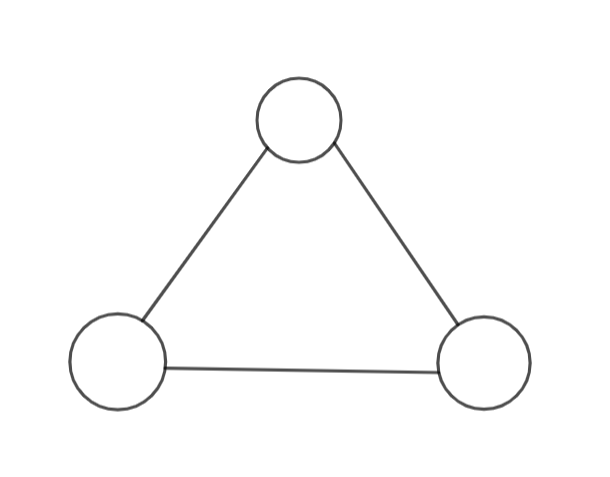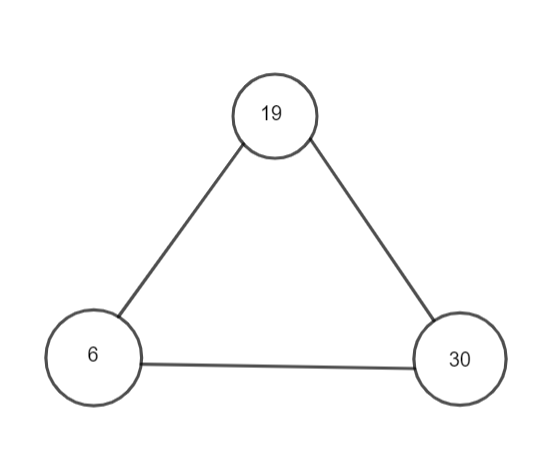Courses
Courses for Kids
Free study material
Offline Centres
MoreLast updated date: 26th Nov 2023
Total views: 277.5k
Views today: 5.77k

# Put three different numbers in the circles so that when you add the numbers at the end of each line you always get a perfect square.Verified
A perfect square is a number that can be expressed as the product of two equal integers. For example, 49 is a perfect square because it is the product of two equal integers, $7\times 7=49$. However, 41 is not a perfect square because it cannot be expressed as the product of two equal integers.
We start by filling one of the circles with 6. Adding 19 to this will give 25 which is a perfect square as $5\times 5=25$. Hence the second circle can be filled with 19. Now, if we add 30 to 19, we get 49 which is again a perfect square as $7\times 7=49$. Also, if 6 and 30 are added then 36 is produced which gives 36 which is also a perfect square since $6\times 6=36$. Hence, the three numbers that can be filled in the circles are 6,19 and 30.